##Director circle of ellipse

The locus of points A, from which the tangents to a fixed ellipse (c), make a right angle at A, is a circle with radius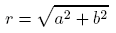Here, a, b, are the major/minor axes of the ellipse. The circle (d) is called [director] circle of the ellipse.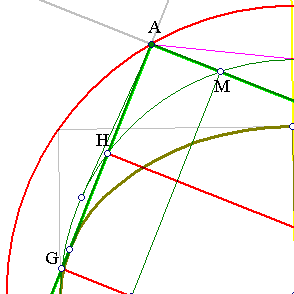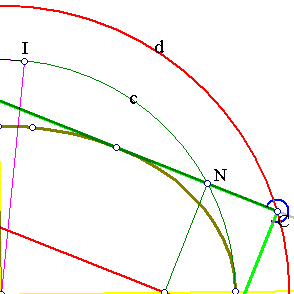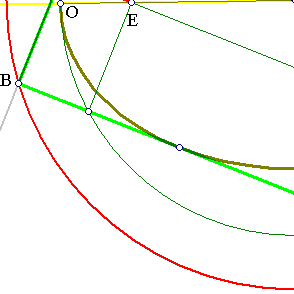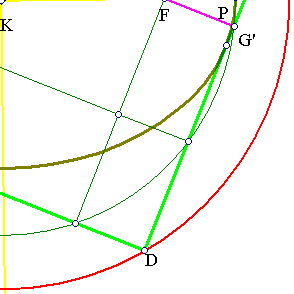The proof follows from the remarks (from the mongraph of Demetrios Bounakis on conic sections, p. 163):
- The foci project on the tangents, to points G, G', H, ... lying on the auxiliary circle c = (K, KI).
- The projections of the foci on the tangent: EG, FH, have |EG||FH| = |FG'||FH| = |FO||FP| = (a+c)(a-c) = b², for c = |EF|/2.
- |AI|² = |AM||AN| = |GE||FH| = b². Hence |AK|² = |AI|²+|IK|² = a²+b² .
For the meaning of the constants, as well as other basic facts on the ellipse, look at Ellipse.html .
Problem: find the locus of points P viewing the ellipse under a fixed angle (phi).

Jacob Steiner (Werke Bd. II, p. 341) gives an interesting application of the director circle to the problem of the determination of the oscillating circle at a point. This is discussed in the file EllipseEvolute.html .

 Produced with EucliDraw© http://users.math.uoc.gr/~pamfilos/